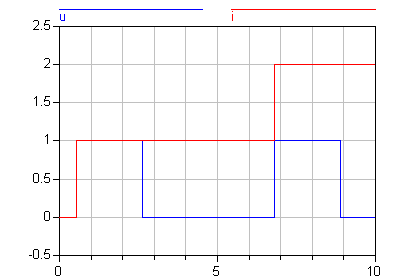# 'edge()'

edge()# Information

This information is part of the Modelica Standard Library maintained by the Modelica Association.

Indicate rising edge

#### Syntax

`edge(b)`

#### Description

Is expanded into "(b and not pre(b))" for Boolean variable b. The same restrictions as for the pre operator apply (e.g., not to be used in function classes).

#### Examples

```model RisingEdge
Boolean u;
Integer i;
equation
u = Modelica.Math.sin(time) > 0.5;
when edge(u) then
i = pre(i) + 1;
end when;
end RisingEdge;```